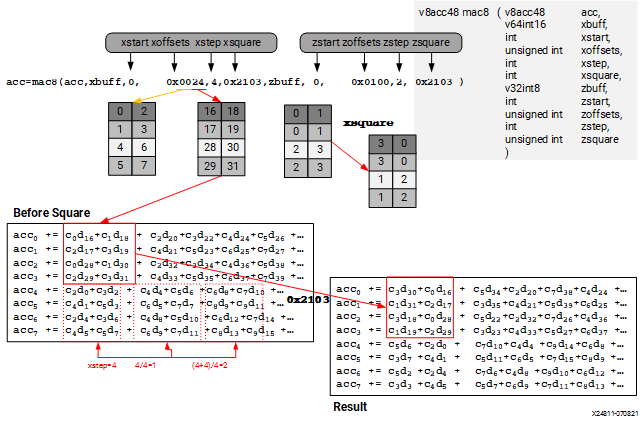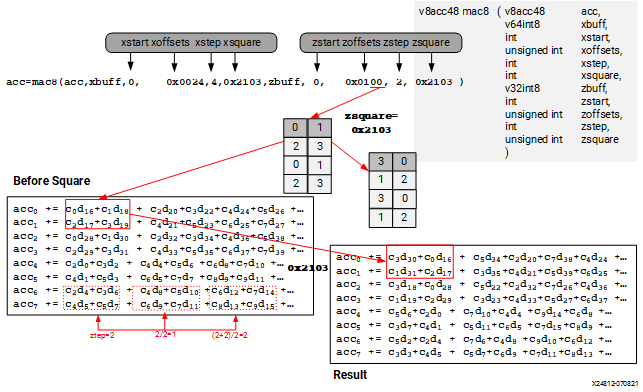# MAC on 8x8 bits - 2022.2 English

## AI Engine Kernel and Graph Programming Guide (UG1079)

Document ID
UG1079
Release Date
2022-10-19
Version
2022.2 English

The following figures show MAC with int8 `X` buffer and int8 `Z` buffer. The first figure shows how data is permuted and the second figure shows how coefficients are permuted. Note that the permute granularity for `X` buffer and `Z` buffer are 32 bits and 16 bits, respectively. The `xoffsets` parameter comes in pair. The first hex value is an absolute 32 bits offset and pick up 4 x 8 bits values (index, index+1, index+2, index+3). The second hex value is offset from the first value + 1 (32 bits offset) and picks up 4 x 8 bits values. For example, `0x00` selects index 0, 1, 2, 3 as well as 4, 5, 6, 7, and `0x24` selects index 16, 17, 18, 19 as well as 28, 29, 30, 31.

There is another `xsquare` parameter to do 8 bits granularity twiddling after main permute. How `xsquare` parameter works in this example can be seen in the center of the following figure.

The `start` (`xstart`, `zstart`) and `step` (`xstep`, `zstep`) parameters are always in terms of data type granularity. Hence, a value of 2 for 16 bits is 2 * 16 bits away, while a value of 2 for 8 bits is 2 * 8 bits away. The `step` parameter applies to the next block of selected data. So, if a pair of `offset` parameters select a 2 * 2 block, the step applies to the next 2 * 2 block. The step added to the index value must be aligned to the permute granularity (32 bits for data, 16 bits for coefficient). For example, when working with 8-bit data, `xstep` needs to be multiples of four. When working with 8-bit coefficient, `zstep` needs to be multiples of two. The following two figures show how `step` works for data and coefficients.

Note that for the coefficient in int8 * int8 types, the 2 * 2 index block is duplicated to construct a 4 * 2 block. See how index 0, 1, 2, and 3 are duplicated in Figure 2.

Figure 1. MAC8 on int8 x int8 Type (X Part)Figure 2. MAC8 on int8 x int8 Type (Z Part)